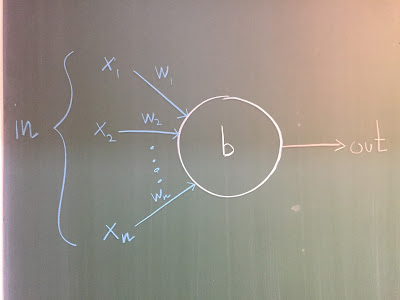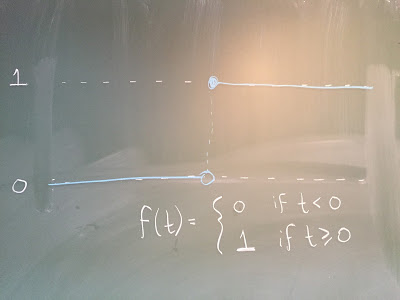# Perceptrons, Neurons, and Learning Logic

OK, fine. I'll tell you about perceptrons.

Warning 1: These are the guys most responsible for getting me hooked on this subject. They may hook you too.

And credit where credit's due: I learned pretty much everything written below from the sections on Perceptrons and Sigmoid Neurons in Chapter 1 of Michael Nielsen's fantastic free on-line Neural Networks and Deep Learning book. In fact, if you'd rather skip my post and just go read those sections now, I won't be offended.

I will, however, insist that afterward you check out the frickin' awesome neural network playground visualization tool created by Daniel Smilkov and Shan Carter as part of this larger project. You can fiddle with various parameters and see in real time how your fiddlings affect the way that a neural net learns to separate various non-linearly separable 2-dimensional data sets. Well worth the 20 minutes of your life it will cost you to do so.

Warning 2: It's going to seem for a moment that we're on a different planet, but bear with me. We'll be back on Earth again soon, I swear.

Perceptrons

Suppose you want to make a Yes/No decision based on your own personal weighting of answers to a finite collection of Yes/No questions.

(I want us all to pause for a moment and appreciate the following fact: This is basically how we do make decisions.)

The example Michael Nielsen gives in his book (Attend a cheese festival: Yes or No?) is far better than anything I can come up with, so I won't try. Instead I'll keep things abstract and just refer you there if you want an example.

A perceptron is a simple mathematical gadget that models this type of decision. People usually draw perceptrons like this:An n-input perceptron

The n nodes on the left (ordered from top to bottom, say, and labeled x1,,xn) represent the inputs. Each input will either be a "0" ("No") or a "1" ("Yes"), so there will be 2n possible inputs to the perceptron. Let's call these inputs x1,,xn.

The output to the perceptron will also be a "0" or "1." How does the perceptron choose? Well, the perceptron has n weights, w1,,wnR, assigned to the n input nodes, along with an overall thresholdbR. Its output is then given by:

{01if ni=1wixi<b,andif ni=1wixib

In other words, if the weighted sum of the inputs achieves or exceeds the threshold, output 1. If it doesn't, output 0.

If you read my post about linearly separating data, this should be starting to sound eerily familiar. Explicitly, a perceptron with n inputs answers the following concrete question:

"Let x⃗ {0,1}nRn be a binary n-tuple, regarded as an n-dimensional real vector. On which side of the hyperplane defined by weight vector w⃗ :=(w1,,wn) and shift b does it lie?"

But when we think about it in these terms, the first thing that should jump to our minds is: Why on earth should a perceptron accept only binary inputs? There's nothing stopping it from accepting real-valued inputs. In fact, the geometric interpretation of what a perceptron is doing is somehow more natural when we allow our inputs to be any real numbers and not just 0s and 1s.

OK. Hold that thought while I tell you something else completely unrelated but even cooler.

Any logical sentence (or "circuit") can be built by concatenating perceptrons:

This is what blew my mind when I first heard about these guys.

If you've ever studied elementary logic (maybe you took an elementary proofs course or something), you've probably built yourself a truth table or two. If you haven't, don't worry. I'll tell you what they are and what they do.

(And if you're interested in what they have to do with mathematical proofs, you might like these notes written by Michael Hutchings, which I often use as a first reading assignment whenever I teach Intro to Proofs at Boston College.)

At its core, elementary logic is a language or "calculus" that allows us to specify how to take a finite, ordered tuple of 0s and 1s (a so-called binary string), perform a collection of operations on it, and obtain another ordered tuple of 0s and 1s. The 4 basic operations in this language are:

1. 1 And 2
2. 1 Or 2
3. If 1 Then 2,
4. Not
The *s above represent inputs. Note that the first three operations take two inputs, and the last takes one.

The binary output or value of each of the statements above is determined by a so-called truth table (it's a bit like a multiplication table). It tells you, e.g., that the output of the statement "1 and 2"is 0 unless both 1 and 2 are 1. This should make sense to you since a statement like "I love cheese and it's 10 AM" is only true if the statements "I love cheese" and "It's 10 AM" are both true.

So the truth table for (1 And 2) looks like this:
The inputs 1 and 2 are along the left and the top, and the outputs for the various corresponding combinations are recorded in the interior. You can easily find the truth tables for the other logical operations online (or in Hutchings' notes), so I won't record those here.

OK, great. The point now is that you can model any of these operations using a perceptron

I'll do "And," then leave the other three as exercises. Here's the picture:
So e.g. if we let w1=w2=10, and b=15, then the perceptron will return 1 iff both inputs are 1, as desired.

Note that there are (infinitely) many choices of weights w1,w2 and shift b that work to model this "And" perceptron; all you need is a hyperplane separating the point (1,1) from the points (0,0),(1,0),(0,1)R2. This is pretty easy to do, and also gives you an idea how to solve the exercises above once you find their truth tables online!

Once you've solved the exercises above, you have all the tinker toys you need to build any simple logical statement out of perceptrons (mathematicians also like using quantifiers like "for all" and "there exists," but let's ignore those). Connect the inputs to outputs in the appropriate way, and you've got your statement. For example, here's a perceptron model of the statement

"If (1 And 2) Then (3)":

For fun, you might now imagine letting 1,2,3 represent the truth values of the statements,
1. "You're happy,"
2. "You know it,"
3. "You clap your hands," resp.
(Credit to Josh Greene for this.)

Then the perceptron circuit will output a 1 if, e.g., you're sad and you don't know it and you clap your hands, but a 0 if you're happy and you know it and you don't clap your hands.

And you can go crazy with it. You can, e.g., make a perceptron circuit that looks like this:

N.B.: It looks like our perceptrons have multiple outputs in this picture. They don't. Each perceptron can have multiple inputs but only a single output. The multiple lines emanating from each perceptron in the pic above correspond to sending that perceptron's output to multiple places.

But I hope that the picture of a perceptron circuit I drew poorly above is starting to look a leeeeeetle bit like the pictures of neural networks we've seen in the news.

And Now Back to That Thought we were Holding:

Perceptron circuits are awesome, but they're pretty rigid. Their inputs and outputs are binary. Not to mention that the rule each perceptron uses to decide between outputting a 0 and a 1 is a step function: output 1 if you're on or to one side of a hyperplane, 0 if you're on the other side.Perceptrons use step functions

The rigidity and jumpiness of this set-up doesn't seem ideally-suited to learning, does it?

(It's not.)

BUT what if we fuzz things out a bit, like we were doing before? That is, let's allow real-valued inputs and outputs, and replace our step functions with sigmoid functions. In other words, let's replace each perceptron with a sigmoid neuron. In case you haven't already guessed, a sigmoid neuron still has associated to it an n-dimensional weight vector w⃗ Rn and a threshold bR. But it allows its n-dimensional input vector x⃗  to live anywhere in Rn, and its output will interpolate between 0 and 1 using a sigmoid function. That is, its output will be σ(w⃗ x⃗ b)(0,1). Recall that σ(t)=11+et.Sigmoid neurons use sigmoid functionsSigmoid functions look an awful lot like step functions if you squint hard at them (increasingly so if we use a sigmoid function like 11+e−ct and let c→∞)

Well, by George, we have a neural network. Moreover, it can, in principle learn logic. At least the elementary models of logic I teach in Math 216.

All we need now is:
1.  a reasonable cost function,
2.  a reasonable way of computing its partial derivatives with respect to the weights and thresholds associated to all of the sigmoid neurons,
3. buttloads of data for it to learn from.
We'll talk more about that next time.

BTW: I should thank the Isaac Newton Institute in Cambridge for its hospitality, since that's where I am at the moment!